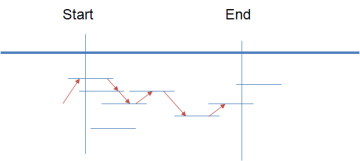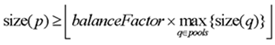# Pipeline Details

When you use the Ion AmpliSeq Designer, the tiling and pooling algorithms run behind the scenes to transform your submitted design into an orderable Custom AmpliSeq kit.

The following sections describe the AmpliSeq tiling and pooling processes:

## The Design Pipeline

The pipeline is optimized to find the highest coverage with the least number of amplicon pools. In an attempt to exhaustively design all primer pairs, the pipeline creates an exhaustive search by sliding a tiling window one-base at a time across the entire targeted region.In creating the primers, the pipeline takes in consideration:

• A basic filtering
• Optimal melting temperatures
• The manufacturability of the resulting primers (the resulting primers should not contain long homopolymers)
• The nucleotide composition should be between 20%-80% GC
• A biological filtering

• SNP filtering: no known SNP (dbSNP 138) is allowed in a primer if its Minor Allele Frequency (MAF) is greater than 5%. If MAF < 5%, SNPs are allowed anywhere in the primer.
• An off-target analysis to minimize off-target hybridization

• An overlap analysis to reduce the number of primers (minimal tiling path), since a higher overlap implies a higher cost

## Tiling Algorithm

The tiling algorithm selects a subset (a tiling) of the input amplicons that meets the following criteria:

• Covers as much of each target sequence as does the original input
• Has many fewer amplicons than the input set
• Maximizes the quality of the amplicons

To do this, the tiling algorithm performs the following steps:

1. Creates an overlap graph of candidate inserts.
2. Assigns a cost to each edge.
3. Returns the tiles from the least-cost path from source to sink.

The output from the tiling algorithm is used as input for the pooling algorithm.

Improvements in the tiling algorithm have significantly increased the in-silico coverage of the designs. The users are enabled to choose between in-silico and assay performance.

## Pooling Algorithm

The pooling algorithm assigns each tile (amplicon) to a pool, subject to requirements that allow each pool to be multiplexed (see "Pooling Requirements", below).

To assign each tile to a pool, the pooling algorithm performs the following steps:

1. Sorts amplicons by decreasing priority.
2. Starts with one available pool.
3. Empties all available pools.
4. For each sorted amplicon:
1. If there is at least one pool in which the amplicon can be multiplexed, the algorithm puts the amplicon into the first compatible pool that maximizes the distance between the amplicon and any other amplicon in the pool.
2. If a new pool can be added, the algorithm adds a new available pool and returns to step 3.
3. If a new pool cannot be added, the algorithm puts the amplicon into the overflow (unpooled) list.

## Pooling Requirements

The following requirements must be met for the pooling algorithm to assign a tile to a pool.

A pool is a set of amplicons meant to be multiplexed (amplified together in a single tube).

• The maximum number of pools is 10.
• The maximum pool capacity is 6144 amplicons.
• The minimum balance factor for every pool p is:• The minimum amplicon separation is 50 bp.

## Advantages of Tiling and Pooling

• The tiling algorithm ensures that the PCR pools cover as much of the targets as do the candidate amplicons supplied to it.
• The pooling algorithm generates a very small number of PCR pools.
• The primers in a pool do not interact.
• Everything runs quickly and with reasonable memory resulting in faster design creation.